Reach Us+44-1474-556909
Two-step Explicit Methods for the Numerical Integration of a System of Second Order Initial Value Problems | OMICS International
Journal of Applied & Computational Mathematics
All submissions of the EM system will be redirected to Online Manuscript Submission System. Authors are requested to submit articles directly to Online Manuscript Submission System of respective journal.

# Two-step Explicit Methods for the Numerical Integration of a System of Second Order Initial Value Problems

Gul MN* and Habib M

Department of Mathematics, University of Engineering and Technology, Lahore, Pakistan

*Corresponding Author:
Gul MN
Department of Mathematics
University of Engineering and Technology
Lahore, Pakistan
Tel: +(92)4299029210
E-mail: [email protected]

Received Date: January 15, 2016; Accepted Date: January 29, 2016; Published Date: February 03, 2016

Citation:Gul MN, Habib M (2016) Two-step Explicit Methods for the Numerical Integration of a System of Second Order Initial Value Problems. J Appl Computat Math 5:286. doi:10.4172/2168-9679.1000286

Copyright: © 2016 Gul MN, et al. This is an open-access article distributed under the terms of the Creative Commons Attribution License, which permits unrestricted use, distribution, and reproduction in any medium, provided the original author and source are credited.

Visit for more related articles at Journal of Applied & Computational Mathematics

#### Abstract

In this paper, authors present a new family of two-step explicit fourth order methods, for the numerical integration of second order periodic initial-value problems. These methods are explicit in nature and we intend to use them, in future, as a predictor for the family of direct hybrid methods. Their stability properties and the efficiency are also discussed. Considering some numerical results, authors saw that the new methods are superior to the existing explicit methods.

#### Keywords

Periodic initial value problem; Stability properties;Interval of periodicity; P-stable

#### Introduction

Authors are mainly concerned with the numerical integration of the special second order initial value systems of order m,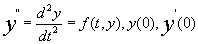given and t>0,            (1)

in which the first derivative does not appear explicitly. Such problems have periodic solutions and they are common in celestial mechanics, quantum mechanical scattering theory, theoretical physics and chemistry, and electronics [1,2]. The direct hybrid methods Cash  and Chawla  are implicit and when applied to a non-linear equation of the form (1), they need a predictor to start the iterations. These predictors should be explicit in nature Gladwell and Thomas  and Wang .

Author describes the basic theory of stability of symmetric multistep methods. Authors develop the explicit fourth order methods. Authors also consider the stability properties. Finally in Section 5, Authors give numerical examples which show that the new explicit methods are more efficient than the other well-known explicit methods, Chawla , , Chawla and Al–Zanaidi , and Franco .

#### Basic Theory

Lambert and Watson  introduce the scalar test equation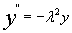(2)

to investigate the stability properties of the methods for solving the initial value problem (1). When authors applied a symmetric twostep method to the scalar test equation (2) they obtained a difference equation of the form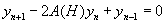(3)

where H =λh, h is the step length,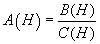where C(H) and B(H) are polynomials in H and yn is the computed approximation to y(nh), n = 0, 1, 2, ….. Following Coleman , when authors apply a symmetric two-step method to the scalar test equation (2) then authors have the difference equation (3). The characteristic equation associated with (3) is given by (4),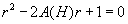(4)

and the roots of the characteristic equation (4) are denoted by r1 and r2.

Definition 1: The numerical method (3) is said to have interval of periodicity, Lambert and Watson .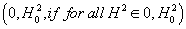, r1 and r2 satisfy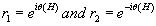(5)

whereθ(H) is a real function of H.

Definition 2:The method (3) is P-stable if its interval of periodicity is (0,∞)Lambert and Watson .

Definition 3:The method (3) is unconditionally stable if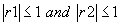for all values ofλh, Hairer .

Theorem 1: A method which has the characteristic equation (4), has an intervalof periodicity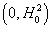, if, for all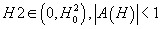.

Having stated the basic theory, authors developed the new explicit methods EXP4, Simos .

#### New Explicit Method

For the numerical integration of the second order initial value problem (1), consider the explicit fourth order method, which the authors will denote as EXP4 and is given by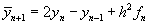(6)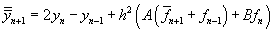(7)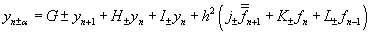(8)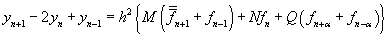(9)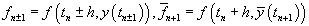,

with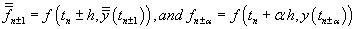,

The method (6-9) is based on the hypotheses that authors put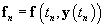and they construct an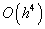order approximation of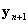. Authors improve this approximation ofby usingorder approximation of. Then, they obtain approximation to yn+α which depend on theorder approximation toand are of order.

Now in order to find the local truncation error (LTE), we expand all the expressions of the method with respect to h around the point yn, as a Taylor series and get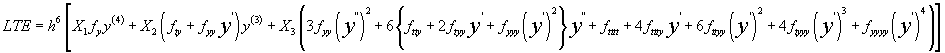(10)

where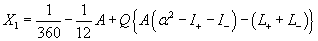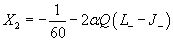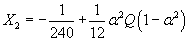Here in (10), we have used subscripts to denoted the partial derivatives, for example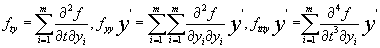and so on. The parameters in the method (6-9) can be chosen freely. But to achieve fourth order accuracy, they must satisfy the conditions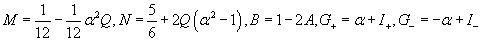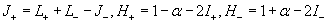(11)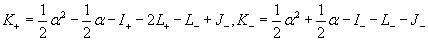So that the parameters α, Q, I+, I-, L+, L-, J- are free. For simplicity, authors take them to be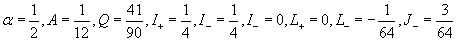(12)

Hence, a particular EXP4 method is(13)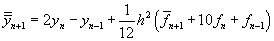(14)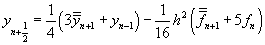(15)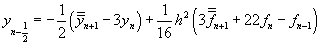(16)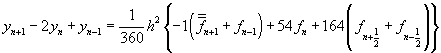(17)

In the next section, we discuss the stability of EXP4.

Stability analysis

To analyze the stability, authors applied the methods (6-9) to the scalar test equation (2) and obtain (3) with

C(H)=1,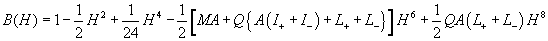Now to find the stability conditions, authors applied the Routh Hurwitz criterion which gives necessary and sufficient conditions for the zeros of a polynomial to have negative real parts to apply thiscriterion, we make the transformation r=(1+Z)/(1-Z), which maps the circle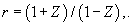which maps the circle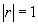into the imaginaryaxis Re(z)=0, the interior of the unit circle into the half plane Re(z)<0and the point r=1 into z=0Lambert . So, authors have, from equation (4).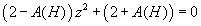Hence the necessary and sufficient conditions for the stability of the methods (6-9) are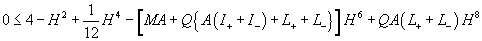(18)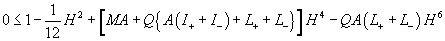When the parameters are given by (12), then the stability conditions (18) give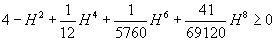(19)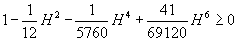Also, it can be easily seen that the stability interval is H∈(0, 2.55). In the next section authors consider a numerical example which shows the method EXP4 performs better than the methods in literature.

#### Numerical Examples

Let us consider the numerical solution of a linear problem, considered by Stiefel and Bettis, Lambert and Watson  and Cash ,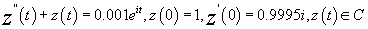(20)

This linear problem has the analytic solution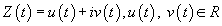where

u(t)=cos(t)+0.0005t sin(t),           (21)

v(t)=sin(t)-0.0005t cos(t)

The analytic solution (21) represents motion on a perturbed circular orbit in the complex z-plane, as shown below in Figure 1.

The distance of the circular orbit from the origin at time t is given by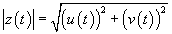(22)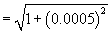Following Lambert and Watson , authors write the complex scalar problem (20) in the equivalent form for u(t)and v(t)as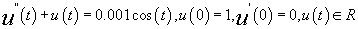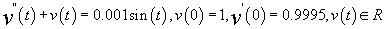The solution to this pair of uncoupled, real, scalar linear problems is computed using the initial conditions at t=0and the analytic solution at t=h, that is we set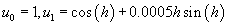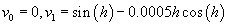Authors obtained the global error at t=40π by comparing the solution obtained by the method \QTR{group}{EXP4} with the analytic solution. Table 1 gives the numerical solutionproduced by the methods (1-4) for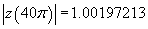obtained with step sizes h=π/2, π/5, π/6, π/9 and π/12 respectively.

h Method 1 Method 2 Method 3 Method 4
π/2 1.00306 1.002003 1.001970 1.001971
π/5 1.002217 1.001981 1.001971 1.001972
π/6 1.002047 1.001975 1.001972 1.001972
π/9 1.001978 1.001972 1.001972 1.001972
π/12 1.001973 1.001972 1.001972 1.001972

Table 1: Comparison of the approximations obtained by Methods 1-4 for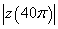.

Where the methods are:

Method 1: Numerov made explicit by Chawla.

Method 2: A two stage fourth order ``almost'' P-stable by Chawla and Al-Zanaidi. Method 3: An explicit hybrid method of Numerov by Franco .

Method 4: Two-step EXP4 method developed in this paper.

On comparing these results, we see that for this problem and for these step sizes, method 4 is more accurate than any of the other methods, especially for larger step sizes. In Table 2, below, authors also present, for comparison purposes, the absolute errors in the computed values ofproduced byusing these methods with step sizes h=π/4, π/8, and π/16 respectively.

h Method 1 Method 2 Method 3 Method 4
π/4 0.101×10-2 0.00314×10-2 0.000219×10-2 0.000100×10-2
π/8 0.123×10-4 0.00507×10-4 0.000245×10-4 0.000134×10-4
π/16 0.180×10-6 0.00823×10-6 0.000170×10-6 0.00020×10-6

Table 2: Comparison of the absolute errors in the appro×imations obtained by Methods 1-4 for.

Table 2 also shows that as the authors halve the step size then the absolute error decreases by a factor of approximately 24. Finally, authors present some conclusions in the next section.

#### Conclusions

Authors have developed explicit fourth order method, which we will be using as a predictor for a class of direct hybrid methods. From the Tables 1 and 2, above, we conclude that the method EXP4 performs much better than the existing methods in the literature and they converge rapidly as compared with other methods. Since the methods based on Numerov usually involve evaluation of first order derivative, which does not appear in the class of problems given byequ(1). So, the methods in literature have a big disadvantage, while our methods EXP4 integrate the equation directly, without requiring the need to calculate the first order derivative.

#### References

Select your language of interest to view the total content in your interested language

### Article Usage

• Total views: 8333
• [From(publication date):
March-2016 - Jul 16, 2019]
• Breakdown by view type
• HTML page views : 8247Can't read the image? click here to refresh